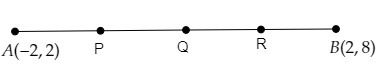# Find the coordinates of the points which divide the line segment joining $A (-2, 2)$ and $B (2, 8)$ into four equal parts.

Given:

The line segment joining the points $A (-2, 2)$ and $B (2, 8)$ is divided into four equal parts.

To do:

We have to find the coordinates of the points which divide the line segment joining the points $A (-2, 2)$ and $B (2, 8)$ in four equal parts.

Solution:

Let $AB$ be a line segment whose ends points are $A (-2, 2)$ and $B (2, 8)$.Let $P, Q, R$ be the points which divide $AB$ in four equal parts.

This implies,

$A P=P Q=Q R=R B$

$Q$ is the mid-point of $\mathrm{AB}$ and $\mathrm{P}$ and $\mathrm{R}$ are the mid points of $A Q$ and $Q B$ respectively.

Using mid-point formula, we get,

The coordinates of $\mathrm{Q}= \left(\frac{-2+2}{2}, \frac{2+8}{2}\right)$

$=\left(\frac{0}{2}, \frac{10}{2}\right)$

$=(0,5)$

The coordinates of $\mathrm{P}= \left(\frac{-2+0}{2}, \frac{2+5}{2}\right)$

$=\left(\frac{-2}{2}, \frac{7}{2}\right)$

$=\left(-1, \frac{7}{2}\right)$

$=(-1,3.5)$

The coordinates of $\mathrm{R}= \left(\frac{0+2}{2}, \frac{5+8}{2}\right)$

$=\left(\frac{2}{2}, \frac{13}{2}\right)$

$=(1,6.5)$

Therefore, the coordinates of the required points are $\left(-1, 3.5\right), (0,5)$ and $(1,6.5)$.

Updated on: 10-Oct-2022

40 Views